# PHP开发APP微信支付接口

### 【准备工作】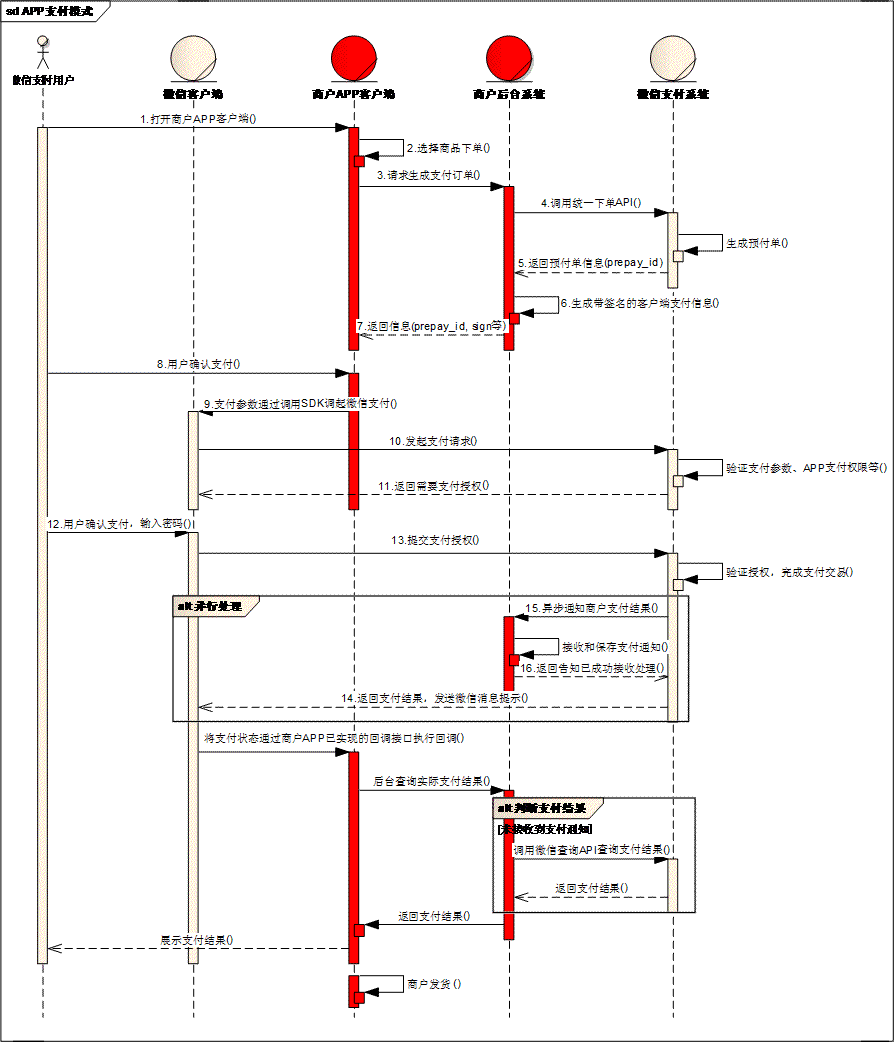APP支付时序图

1. 用户在商户APP中选择商品，提交订单，选择微信支付。
2. 商户后台收到用户支付单，调用微信支付统一下单接口。参见 【统一下单API】
3. 统一下单接口返回正常的prepay_id，再按签名规范重新生成签名后，将数据传输给APP。参与签名的字段名为appId，partnerId，prepayId，nonceStr，timeStamp，package。注意：package的值格式为Sign=WXPay
4. 商户APP调起微信支付。api参见本章节 【app端开发步骤说明】
5. 商户后台接收支付通知。api参见 【支付结果通知API】
6. 商户后台查询支付结果。api参见 【查询订单API】

### 【着手开发】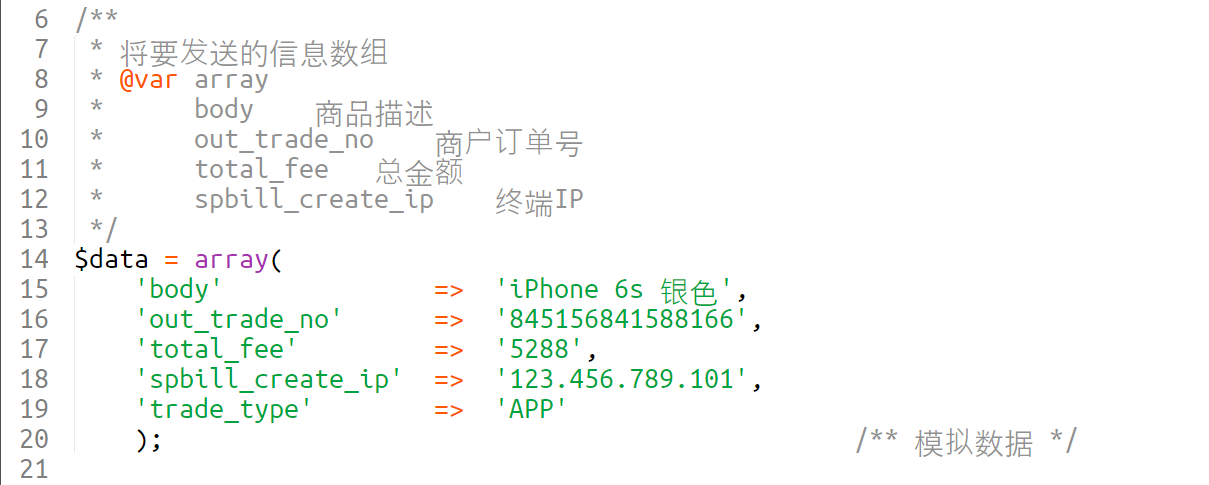appid 和 mch_id 分别去到微信开放平台和微信商户平台中获取，nonce_str (随机字符串) 很随意了，不长于32位就好。


/**
* 生成随机数并返回
*/
private function getNonceStr() {
$code = ""; for ($i=0; $i > 10;$i++) {
$code .= mt_rand(1000); //获取随机数 }$nonceStrTemp = md5($code);$nonce_str = mb_substr($nonceStrTemp, 5,37); //MD5加密后截取32位字符 return$nonce_str;
}

notify_url（通知地址）是接收微信支付异步通知回调地址，通知url必须为直接可访问的url，不能携带参数。例如：'https://pay.weixin.qq.com/wxpay/pay.action'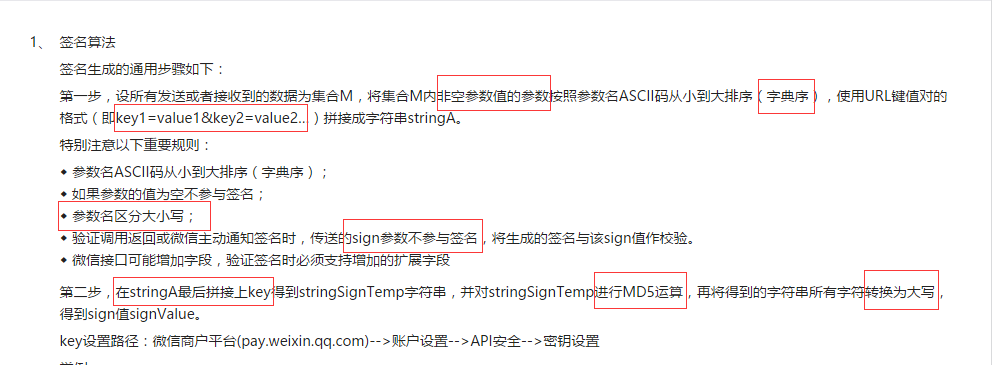/**
* 获取参数签名；
* @param  Array  要传递的参数数组
* @return String 通过计算得到的签名；
*/
private function getSign($params) { ksort($params);        //将参数数组按照参数名ASCII码从小到大排序
foreach ($params as$key => $item) { if (!empty($item)) {         //剔除参数值为空的参数
$newArr[] =$key.'='.$item; // 整合新的参数数组 } }$stringA = implode("&", $newArr); //使用 & 符号连接参数$stringSignTemp = $stringA."&key=".$this->key;        //拼接key
// key是在商户平台API安全里自己设置的
$stringSignTemp = MD5($stringSignTemp);       //将字符串进行MD5加密
$sign = strtoupper($stringSignTemp);      //将所有字符转换为大写
return $sign; } 注意：key的值长度不能超过32位。 这里，我们最好编写一个类文件来包含这些方法，比如上面我们获取签名的方法会重复调用很多次，写在类方法里能减少耦合，并且方便多次调用。 那么我编写了一个微信支付的类文件（我会在文章的最末尾将源码提供给大家参考），该类在实例化的同时会初始化一些固定数据，例如appid 、 mch_id 等  /** * 构造函数，初始化成员变量 * @param String$appid  商户的应用ID
* @param  Int $mch_id 商户编号 * @param String$key 秘钥
*/
// 将构造函数设置为私有，禁止用户实例化该类
private function __construct($appid,$mch_id, $key) { if (is_string($appid) && is_string($mch_id)) {$this->appid = $appid;$this->mch_id = $mch_id;$this->key = $key; } } /** * 获取微信支付类实例 * 该类使用单例模式 * @return WeEncryption 本类实例 */ public static function getInstance() { if(self::$instance == null) {
self::$instance = new Self(APPID, MCHID, APP_KEY); } return self::$instance;
}

*/
public function setNotifyUrl($url) { if (is_string($url)) {
$this->notify_url =$url;
}
}

/**
* 拼装请求的数据
* @return  String 拼装完成的数据
*/
private function setSendData($data) {$this->sTpl = "<xml>
<appid><![CDATA[%s]]></appid>
<body><![CDATA[%s]]></body>
<mch_id><![CDATA[%s]]></mch_id>
<nonce_str><![CDATA[%s]]></nonce_str>
<notify_url><![CDATA[%s]]></notify_url>
<spbill_create_ip><![CDATA[%s]]></spbill_create_ip>
<total_fee><![CDATA[%d]]></total_fee>
<sign><![CDATA[%s]]></sign>
</xml>";                          //xml数据模板

$nonce_str =$this->getNonceStr();        //调用随机字符串生成方法获取随机字符串

$data['appid'] =$this->appid;
$data['mch_id'] =$this->mch_id;
$data['nonce_str'] =$nonce_str;
$data['notify_url'] =$this->notify_url;
$data['trade_type'] =$this->trade_type;      //将参与签名的数据保存到数组
// 注意：以上几个参数是追加到$data中的，$data中应该同时包含开发文档中要求必填的剔除sign以外的所有数据
$sign =$this->getSign($data); //获取签名$data = sprintf($this->sTpl,$this->appid, $data['body'],$this->mch_id, $nonce_str,$this->notify_url, $data['out_trade_no'],$data['spbill_create_ip'], $data['total_fee'],$this->trade_type, $sign); //生成xml数据格式 return$data;
}

• xml数据要使用<![CDATA[]]>注释包裹


/**
* 发送下单请求；
* @param  Curl   $curl 请求资源句柄 * @return mixed 请求返回数据 */ public function sendRequest(Curl$curl, $data) {$data = $this->setSendData($data);            //获取要发送的数据
$url = "https://api.mch.weixin.qq.com/pay/unifiedorder";$curl->setUrl($url); //设置请求地址$content = $curl->execute(true, 'POST',$data);       //执行该请求
return $content; //返回请求到的数据 } 以上示例代码中包含了一个Curl类，是一个数据请求工具类，不了解的小伙伴可以百度查一下。该工具主要是帮助我们发送请求用的，稍后我会在文章的最后将该类文件的源码跟微信支付类一起展示给大家。 我们在客户端代码中实例化该工具类，调用 WeEncryption::sendRequest(Curl$curl, $data) 方法请求下单接口： $curl = new Curl();                //实例化传输类；
$xml_data =$encpt->sendRequest($curl,$data);        //发送请求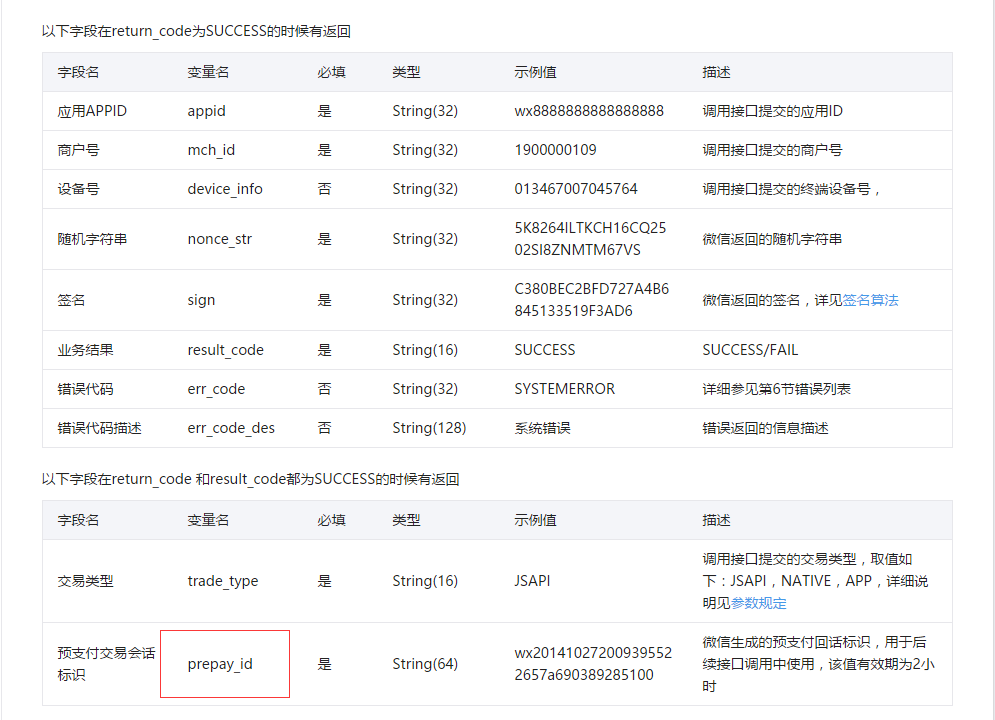• 统一下单接口返回正常的prepay_id，再按签名规范重新生成签名后，将数据传输给APP。
• 参与签名的字段名为appId，partnerId，prepayId，nonceStr，timeStamp，package。

if ($postObj === false) { echo 'FAIL'; exit; // 如果解析的结果为false，终止程序 } if ($postObj->return_code == 'FAIL') {
echo $postObj->return_msg; // 如果微信返回错误码为FAIL，则代表请求失败，返回失败信息； } else { //如果上一次请求成功，那么我们将返回的数据重新拼装，进行第二次签名$resignData = array(
'appid'    =>    $postObj->appid, 'partnerId' =>$postObj->mch_id,
'prepayId'    =>    $postObj->prepay_id, 'nonceStr' =>$postObj->nonce_str,
'timeStamp'    =>    time(),
'package'    =>    'Sign=WXPay'
);
//二次签名；
$sign =$encpt->getClientPay($resignData); echo$sign;
}

* @return Object         返回Obj对象
*/
public function xmlToObject($xmlStr) { if (!is_string($xmlStr) || empty($xmlStr)) { return false; } // 由于解析xml的时候，即使被解析的变量为空，依然不会报错，会返回一个空的对象，所以，我们这里做了处理，当被解析的变量不是字符串，或者该变量为空，直接返回false$postObj = simplexml_load_string($xmlStr, 'SimpleXMLElement', LIBXML_NOCDATA);$postObj = json_decode(json_encode($postObj)); //将xml数据转换成对象返回 return$postObj;
}

• package的值为Sign=WXPay不变
• 时间戳使用time()获取就好
• mch_id 即为 partnerId
• 其他数据可以使用微信返回的数据，也可以自己写
• 最重要的一点，看下图：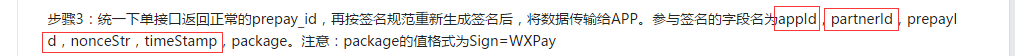* @return String       签名字符串
*/
public function getClientPay($data) {$sign = $this->getSign($data);        // 生成签名并返回
return $sign; } 将重新生成的签名传输给 APP 客户端。 返回的时候，要将sign，appId，partnerId，prepayId，nonceStr，timeStamp，package 这七个值一起返回个 APP 客户端。 ### 【验签】 完成前面我们讲解的过程之后，APP客户端已经可以调起微信的支付界面进行支付了，但是整个过程还没有完成。为了用户资金的安全起见，防止数据被篡改，我们要对微信返回过来的数据进行验证。 还记得我们上一次向微信发送请求的时候，我们填写了一个 notify_url 的参数吗？当APP客户端请求支付成功后，微信会发起一个并行操作： 1. 向APP客户端返回支付状态 2. 向商户后台服务器返回支付结果 我们先来获取一下微信向商户后台服务器返回的结果：  /** * 接收支付结果通知参数 * @return Object 返回结果对象； */ public function getNotifyData() {$postXml = $GLOBALS["HTTP_RAW_POST_DATA"]; // 接受通知参数； if (empty($postXml)) {
return false;
}
$postObj =$this->xmlToObject($postXml); // 调用解析方法，将xml数据解析成对象 if ($postObj === false) {
return false;
}
if (!empty($postObj->return_code)) { if ($postObj->return_code == 'FAIL') {
return false;
}
}
return $postObj; // 返回结果对象； } 然后我们在客户端代码中接收一下： $obj = $encpt->getNotifyData(); // 接收数据对象 然后重新拼装数据准备第三次签名：  if ($obj) {
$data = array( 'appid' =>$obj->appid,
'mch_id'            =>    $obj->mch_id, 'nonce_str' =>$obj->nonce_str,
'result_code'        =>    $obj->result_code, 'openid' =>$obj->openid,
'trade_type'        =>    $obj->trade_type, 'bank_type' =>$obj->bank_type,
'total_fee'            =>    $obj->total_fee, 'cash_fee' =>$obj->cash_fee,
'transaction_id'    =>    $obj->transaction_id, 'out_trade_no' =>$obj->out_trade_no,
'time_end'            =>    $obj->time_end ); // 拼装数据进行第三次签名$sign = $encpt->getSign($data);        // 获取签名

/** 将签名得到的sign值和微信传过来的sign值进行比对，如果一致，则证明数据是微信返回的。 */
if ($sign ==$obj->sign) {
$reply = "<xml> <return_code><![CDATA[SUCCESS]]></return_code> <return_msg><![CDATA[OK]]></return_msg> </xml>"; echo$reply;      // 向微信后台返回结果。
exit;
}
}


/**
* 查询订单状态
* @param  Curl   $curl 工具类 * @param string$out_trade_no 订单号
* @return xml               订单查询结果
*/
public function queryOrder(Curl $curl,$out_trade_no) {
$nonce_str =$this->getNonceStr();
$data = array( 'appid' =>$this->appid,
'mch_id'    =>    $this->mch_id, 'out_trade_no' =>$out_trade_no,
'nonce_str'            =>    $nonce_str );$sign = $this->getSign($data);
$xml_data = '<xml> <appid>%s</appid> <mch_id>%s</mch_id> <nonce_str>%s</nonce_str> <out_trade_no>%s</out_trade_no> <sign>%s</sign> </xml>';$xml_data = sprintf($xml_data,$this->appid, $this->mch_id,$nonce_str, $out_trade_no,$sign);
$url = "https://api.mch.weixin.qq.com/pay/orderquery";$curl->setUrl($url);$content = $curl->execute(true, 'POST',$xml_data);
return \$content;
}

### 【结束】